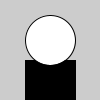# create_shape()#

The create_shape() function is used to define a new shape.

## Examples#def setup():
global s  # the Py5Shape object
# creating the Py5Shape as a square. the
# numeric arguments are similar to rect().
s = py5.create_shape(py5.RECT, 0, 0, 50, 50)
s.set_fill("#0000FF")
s.set_stroke(False)

def draw():
py5.shape(s, 25, 25)def setup():
global s  # the Py5Shape object
# creating a custom Py5Shape as a square, by
# specifying a series of vertices.
s = py5.create_shape()
s.begin_shape()
s.fill(0, 0, 255)
s.no_stroke()
s.vertex(0, 0)
s.vertex(0, 50)
s.vertex(50, 50)
s.vertex(50, 0)
s.end_shape(py5.CLOSE)

def draw():
py5.shape(s, 25, 25)def setup():
global s
py5.size(100, 100, py5.P2D)
s = py5.create_shape()
s.begin_shape(py5.TRIANGLE_STRIP)
s.vertex(30, 75)
s.vertex(40, 20)
s.vertex(50, 75)
s.vertex(60, 20)
s.vertex(70, 75)
s.vertex(80, 20)
s.vertex(90, 75)
s.end_shape()

def draw():
py5.shape(s, 0, 0)def setup():
# create the shape group
global alien
alien = py5.create_shape(py5.GROUP)

# make two shapes
py5.ellipse_mode(py5.CORNER)
head = py5.create_shape(py5.ELLIPSE, -25, 0, 50, 50)
body = py5.create_shape(py5.RECT, -25, 45, 50, 40)
body.set_fill("#000000")

# add the two "child" shapes to the parent group

def draw():
py5.background(204)
py5.translate(50, 15)
py5.shape(alien)  # draw the group


## Description#

The create_shape() function is used to define a new shape. Once created, this shape can be drawn with the shape() function. The basic way to use the function defines new primitive shapes. One of the following parameters are used as the first parameter: ELLIPSE, RECT, ARC, TRIANGLE, SPHERE, BOX, QUAD, or LINE. The parameters for each of these different shapes are the same as their corresponding functions: ellipse(), rect(), arc(), triangle(), sphere(), box(), quad(), and line(). The first example clarifies how this works.

Custom, unique shapes can be made by using create_shape() without a parameter. After the shape is started, the drawing attributes and geometry can be set directly to the shape within the begin_shape() and end_shape() methods. See the second example for specifics, and the reference for begin_shape() for all of its options.

The create_shape() function can also be used to make a complex shape made of other shapes. This is called a “group” and it’s created by using the parameter GROUP as the first parameter. See the fourth example to see how it works.

After using create_shape(), stroke and fill color can be set by calling methods like Py5Shape.set_fill() and Py5Shape.set_stroke(), as seen in the examples. The complete list of methods and fields for the Py5Shape class are in the py5 documentation.

Underlying Processing method: createShape

## Signatures#

create_shape() -> Py5Shape

create_shape(
kind: int,  # either POINT, LINE, TRIANGLE, QUAD, RECT, ELLIPSE, ARC, BOX, SPHERE
/,
*p: float,
) -> Py5Shape

create_shape(
type: int,  # either GROUP, PATH, or GEOMETRY
/,
) -> Py5Shape


Updated on March 06, 2023 02:49:26am UTC